# SCC Education

## Oxidation number,Types of Oxidation-Reduction Reactions

Oxidation number
The charge the atom would have in a molecule (or an ionic compound) if electrons were completely transferred.
1.Free elements (un-combined state) have an oxidation number of zero.
Na, Be, K, Pb, H2, O2, P4  = 0
2.In mono atomic ions, the oxidation number is equal to the charge on the ion.
Li+, Li = +1; Fe3+, Fe = +3;  O2-, O = -2
3.The oxidation number of oxygen is usually –2.  In H2O2 and O22- it is  –1
4.The oxidation number of hydrogen is +1 except when it
is bonded to metals in binary compounds.  In these
cases, its oxidation number is –1.
5.Group IA metals are +1, IIA metals are +2 and fluorine
is always –1.
6.  The sum of the oxidation numbers of all the atoms in a molecule or ion is equal to the charge on the molecule or ion.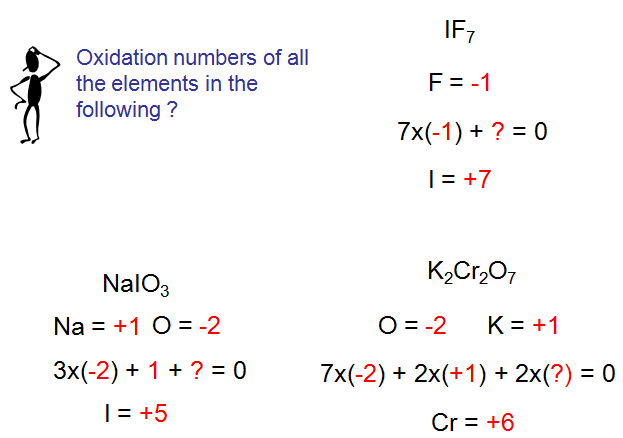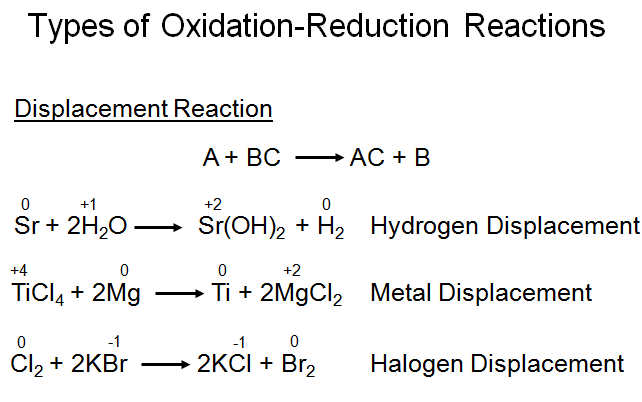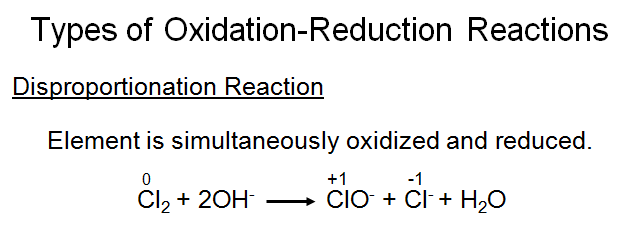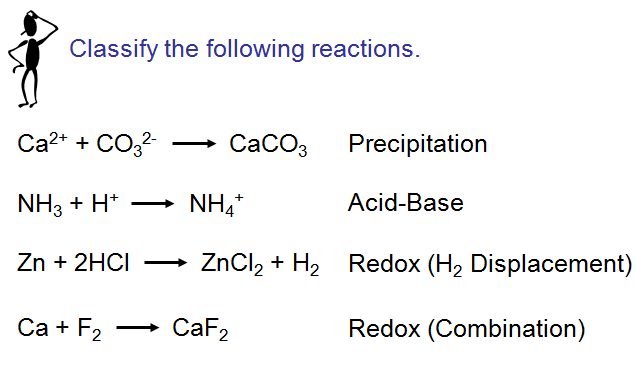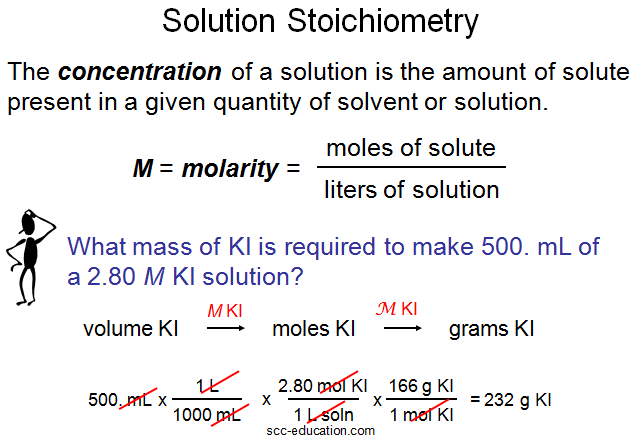Chemistry for class 12 ...........